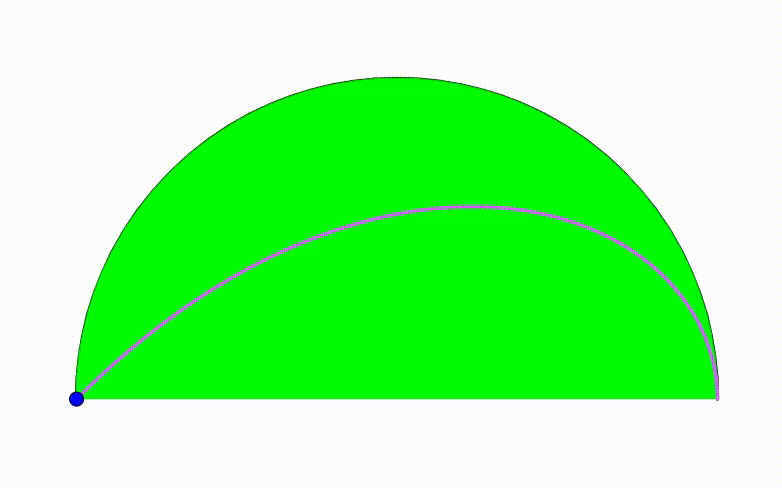# Dynamic Geometry: P89

Geometry Level pendingThe diagram shows a black semicircle. The cyan and green semicircles are tangent to each other and internally tangent to the black semicircle. They are growing and shrinking freely so that the sum of their radius is always equal to $1$. We draw a red vertical segment using their tangency point. At last we inscribed two yellow circles so they are tangent to the red line, to the black semicircle and to one of the two bottom semicircles. The tangency point between the cyan circle and one yellow circle traces a locus (purple curve). The ratio of the area bounded by the purple curve and the black semicirle's diameter to the area of the black semicircle can be expressed as $\dfrac{p}{\pi }+q$, where $p$ and $q$ are integers. Find $p+q$.

×